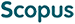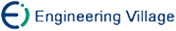郭育生, 黄 磊, 刘昌平. 基于多候选的数学公式识别系统[J]. 计算机研究与发展, 2007, 44(7): 1144-1150.
 引用本文: 郭育生, 黄 磊, 刘昌平. 基于多候选的数学公式识别系统[J]. 计算机研究与发展, 2007, 44(7): 1144-1150.Guo Yusheng, Huang Lei, Liu Changping. A Multi-Candidate Mathematical Expression Recognition System[J]. Journal of Computer Research and Development, 2007, 44(7): 1144-1150.
 Citation: Guo Yusheng, Huang Lei, Liu Changping. A Multi-Candidate Mathematical Expression Recognition System[J]. Journal of Computer Research and Development, 2007, 44(7): 1144-1150.## A Multi-Candidate Mathematical Expression Recognition System

• 摘要: 提出了一种基于多候选方法的数学公式识别系统.该系统主要包括公式图像预处理,多候选公式符号分割和多候选公式结构分析3个部分.在公式符号切分中，使用3次动态规划方法对公式图像进行多候选公式符号切分.在公式结构分析中，采用层次结构方法多候选分析公式符号间的结构关系，然后使用LaTex格式和MathType格式表示数学公式的识别结果.为了确定符号间的空间位置关系,建立了符号的空间关系模型.在3268个公式图像组成的测试集上取得了78.2%的公式分析正确率.

Abstract: A multi-candidate mathematical expression (ME) recognition system is proposed. The system includes three main components: image preprocessing, symbol segmentation and structure analysis. During the symbol segmentation period, a three-stage segmentation method based on dynamic programming (DP) is proposed. In the initial segmentation based on DP algorithm, the ME image is segmented into several blocks. In the vertical segmentation, each block is segmented into some blobs. In the horizontal segmentation based on DP algorithm, every blob is segmented into symbols. During the structure analysis period, hierarchical structure is adopted to analyze structure of ME. The hierarchical structure analysis method consists of three steps, i.e., matrix analysis, sub-expression analysis and script expression analysis. In matrix analysis (sub-expression analysis), an ME is decomposed into several basic matrixes (basic sub-expressions) and some sub-expressions (script expressions) by reconstructing the ME (sub-expression) global structure, and then every basic matrix (sub-expression) is analyzed from bottom to up. In script analysis, a graph rewriting algorithm is adopted to build script relation trees among symbols within a script expression. A spatial relation model is built to calculate spatial relations' confidence between two symbols. The experiments are implemented on a database with 3268 ME images and the results show that the proposed system works well. Top-1 ME recognition accuracy reaches 78.2%./下载:  全尺寸图片 幻灯片
• 分享
• 用微信扫码二维码

分享至好友和朋友圈